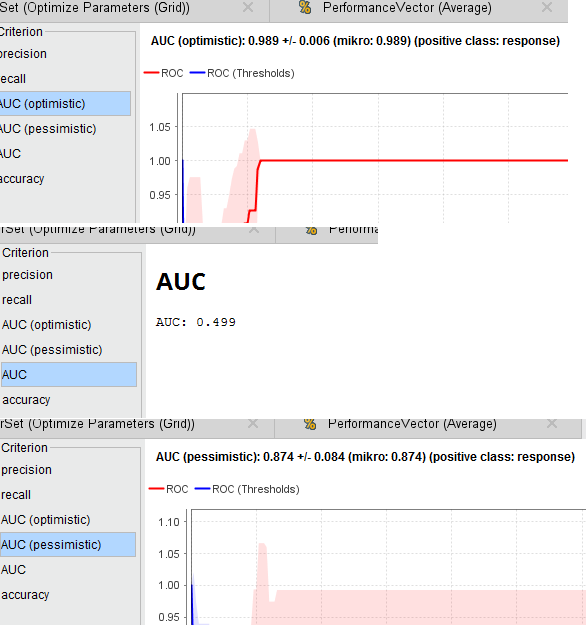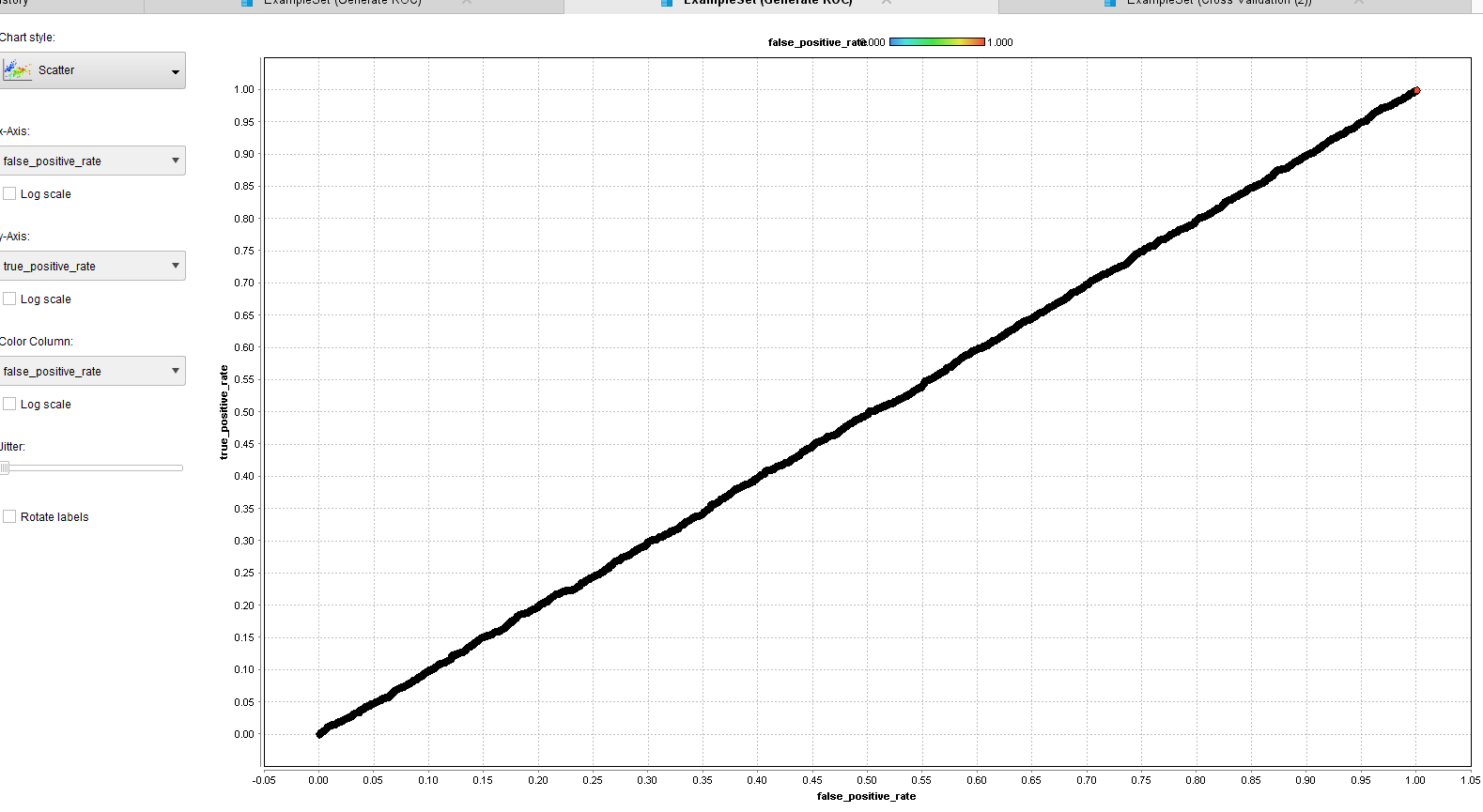# How to interpret this surprising AUC result ?

Moderator, RapidMiner Certified Analyst, Member Posts: 1,195Unicorn
edited June 2019 in Help

Dear all,

During my experimentations, I builded a "classic" process with a model (Random Forest) inside a X-validation operator, inside an Optimize Parameters operator.

After running, I found surprising results :

1. AUC << AUC(pessimistic) < AUC (optimistic)l

2. The curve ROC associated to AUC is absent !

I used the Generate ROC operator to represent the ROC curve and the shape of this curve is compatible

with the calculated value of AUC (0.499) :Questions :

- How to interpret this result ?

- Why the ROC curve associated to AUC from the Performance operator is absent ?

- What are the definitions of AUC (pessimistic) / AUC (optimistic) ?

Regards,

Lionel

NB : The process :

`<?xml version="1.0" encoding="UTF-8"?><process version="8.2.000">  <context>    <input/>    <output/>    <macros/>  </context>  <operator activated="true" class="process" compatibility="8.2.000" expanded="true" name="Process">    <process expanded="true">      <operator activated="true" class="generate_direct_mailing_data" compatibility="8.2.000" expanded="true" height="68" name="Generate Direct Mailing Data" width="90" x="45" y="187">        <parameter key="number_examples" value="10000"/>      </operator>      <operator activated="true" class="split_data" compatibility="8.2.000" expanded="true" height="103" name="Split Data" width="90" x="246" y="136">        <enumeration key="partitions">          <parameter key="ratio" value="0.8"/>          <parameter key="ratio" value="0.2"/>        </enumeration>      </operator>      <operator activated="true" class="concurrency:optimize_parameters_grid" compatibility="8.2.000" expanded="true" height="145" name="Optimize Parameters (Grid)" width="90" x="380" y="85">        <list key="parameters">          <parameter key="Random Forest.number_of_trees" value="[2;5;10;linear]"/>          <parameter key="Random Forest.maximal_depth" value="[2;5;10;linear]"/>        </list>        <process expanded="true">          <operator activated="true" class="concurrency:cross_validation" compatibility="8.2.000" expanded="true" height="145" name="Cross Validation (2)" width="90" x="112" y="85">            <process expanded="true">              <operator activated="true" class="concurrency:parallel_random_forest" compatibility="8.2.000" expanded="true" height="103" name="Random Forest" width="90" x="179" y="34"/>              <connect from_port="training set" to_op="Random Forest" to_port="training set"/>              <connect from_op="Random Forest" from_port="model" to_port="model"/>              <portSpacing port="source_training set" spacing="0"/>              <portSpacing port="sink_model" spacing="0"/>              <portSpacing port="sink_through 1" spacing="0"/>            </process>            <process expanded="true">              <operator activated="true" class="apply_model" compatibility="8.2.000" expanded="true" height="82" name="Apply Model (2)" width="90" x="112" y="85">                <list key="application_parameters"/>              </operator>              <operator activated="true" class="performance" compatibility="8.2.000" expanded="true" height="82" name="Performance Training" width="90" x="224" y="85"/>              <connect from_port="model" to_op="Apply Model (2)" to_port="model"/>              <connect from_port="test set" to_op="Apply Model (2)" to_port="unlabelled data"/>              <connect from_op="Apply Model (2)" from_port="labelled data" to_op="Performance Training" to_port="labelled data"/>              <connect from_op="Performance Training" from_port="performance" to_port="performance 1"/>              <connect from_op="Performance Training" from_port="example set" to_port="test set results"/>              <portSpacing port="source_model" spacing="0"/>              <portSpacing port="source_test set" spacing="0"/>              <portSpacing port="source_through 1" spacing="0"/>              <portSpacing port="sink_test set results" spacing="0"/>              <portSpacing port="sink_performance 1" spacing="0"/>              <portSpacing port="sink_performance 2" spacing="0"/>            </process>          </operator>          <connect from_port="input 1" to_op="Cross Validation (2)" to_port="example set"/>          <connect from_op="Cross Validation (2)" from_port="model" to_port="model"/>          <connect from_op="Cross Validation (2)" from_port="test result set" to_port="output 1"/>          <connect from_op="Cross Validation (2)" from_port="performance 1" to_port="performance"/>          <portSpacing port="source_input 1" spacing="0"/>          <portSpacing port="source_input 2" spacing="0"/>          <portSpacing port="sink_performance" spacing="0"/>          <portSpacing port="sink_model" spacing="0"/>          <portSpacing port="sink_output 1" spacing="0"/>          <portSpacing port="sink_output 2" spacing="0"/>        </process>      </operator>      <operator activated="true" class="anomalydetection:Generate ROC" compatibility="2.4.001" expanded="true" height="145" name="Generate ROC" width="90" x="581" y="34">        <parameter key="label value for outliers" value="response"/>      </operator>      <operator activated="true" class="concurrency:loop" compatibility="8.2.000" expanded="true" height="103" name="Loop" width="90" x="581" y="187">        <parameter key="number_of_iterations" value="20"/>        <process expanded="true">          <operator activated="true" class="sample" compatibility="8.2.000" expanded="true" height="82" name="Sample" width="90" x="45" y="136">            <parameter key="sample" value="relative"/>            <list key="sample_size_per_class"/>            <list key="sample_ratio_per_class"/>            <list key="sample_probability_per_class"/>            <parameter key="use_local_random_seed" value="true"/>            <parameter key="local_random_seed" value="%{iteration}"/>            <description align="center" color="transparent" colored="false" width="126">Random Subsets of test data. Random Seed is based on iteration.</description>          </operator>          <operator activated="true" class="apply_model" compatibility="8.2.000" expanded="true" height="82" name="Apply Model (4)" width="90" x="179" y="34">            <list key="application_parameters"/>          </operator>          <operator activated="true" class="performance" compatibility="8.2.000" expanded="true" height="82" name="Performance Testing" width="90" x="380" y="34"/>          <connect from_port="input 1" to_op="Apply Model (4)" to_port="model"/>          <connect from_port="input 2" to_op="Sample" to_port="example set input"/>          <connect from_op="Sample" from_port="example set output" to_op="Apply Model (4)" to_port="unlabelled data"/>          <connect from_op="Apply Model (4)" from_port="labelled data" to_op="Performance Testing" to_port="labelled data"/>          <connect from_op="Performance Testing" from_port="performance" to_port="output 1"/>          <portSpacing port="source_input 1" spacing="0"/>          <portSpacing port="source_input 2" spacing="0"/>          <portSpacing port="source_input 3" spacing="0"/>          <portSpacing port="sink_output 1" spacing="0"/>          <portSpacing port="sink_output 2" spacing="0"/>        </process>      </operator>      <operator activated="true" breakpoints="after" class="average" compatibility="8.2.000" expanded="true" height="82" name="Average" width="90" x="715" y="187"/>      <operator activated="true" class="t_test" compatibility="8.2.000" expanded="true" height="124" name="T-Test" width="90" x="849" y="136"/>      <connect from_op="Generate Direct Mailing Data" from_port="output" to_op="Split Data" to_port="example set"/>      <connect from_op="Split Data" from_port="partition 1" to_op="Optimize Parameters (Grid)" to_port="input 1"/>      <connect from_op="Split Data" from_port="partition 2" to_op="Loop" to_port="input 2"/>      <connect from_op="Optimize Parameters (Grid)" from_port="performance" to_op="Generate ROC" to_port="performance"/>      <connect from_op="Optimize Parameters (Grid)" from_port="model" to_op="Loop" to_port="input 1"/>      <connect from_op="Optimize Parameters (Grid)" from_port="parameter set" to_port="result 4"/>      <connect from_op="Optimize Parameters (Grid)" from_port="output 1" to_op="Generate ROC" to_port="example set"/>      <connect from_op="Generate ROC" from_port="example set" to_port="result 5"/>      <connect from_op="Generate ROC" from_port="roc set" to_port="result 6"/>      <connect from_op="Generate ROC" from_port="auc" to_port="result 7"/>      <connect from_op="Generate ROC" from_port="performance" to_op="T-Test" to_port="performance 1"/>      <connect from_op="Loop" from_port="output 1" to_op="Average" to_port="averagable 1"/>      <connect from_op="Average" from_port="average" to_op="T-Test" to_port="performance 2"/>      <connect from_op="T-Test" from_port="significance" to_port="result 1"/>      <connect from_op="T-Test" from_port="performance 1" to_port="result 2"/>      <connect from_op="T-Test" from_port="performance 2" to_port="result 3"/>      <portSpacing port="source_input 1" spacing="0"/>      <portSpacing port="sink_result 1" spacing="0"/>      <portSpacing port="sink_result 2" spacing="0"/>      <portSpacing port="sink_result 3" spacing="0"/>      <portSpacing port="sink_result 4" spacing="0"/>      <portSpacing port="sink_result 5" spacing="0"/>      <portSpacing port="sink_result 6" spacing="0"/>      <portSpacing port="sink_result 7" spacing="0"/>      <portSpacing port="sink_result 8" spacing="0"/>      <portSpacing port="sink_result 9" spacing="0"/>    </process>  </operator></process>`

Tagged:

• Moderator, RapidMiner Certified Analyst, Member Posts: 1,195Unicorn

Hi again,

To follow my previous post :

For those who, like me, ask the following question :

"What are the definitions of AUC (pessimistic) / AUC (optimistic) ?"

Here the documentation  of RapidMiner about the Performance Binomial Classification operator.

According to this document "The normal version of AUC calculates the area by taking the average of AUC (optimistic) and AUC (pessimistic)"

==> This definition is a priori imcompatible with the results given in the previous post.

I hope this mystery will dissipate...

Regards,

Lionel

• Moderator, RapidMiner Certified Analyst, RapidMiner Certified Expert, Member Posts: 1,635Unicorn

Hi @lionelderkrikor, well I think I know what the definition of AUC (pessimistic) vs (optimistic) is SUPPOSED to be, but given your results, there may be a bug--at least in certain cases.

The pessimistic version is is supposed to resolve score ties in the least favorable way in terms of correct classification (e.g., all the wrong classifications get sorted first), and the optimistic version is supposed to resolve those same ties in the most favorable way (e.g., the correct classifications get sorted first).  The normal AUC then logically is splitting the difference (which should be the same as randomly ordering the ties).

Brian T.
Lindon Ventures
Data Science Consulting from Certified RapidMiner Experts
• Moderator, RapidMiner Certified Analyst, Member Posts: 1,195Unicorn

Ok, I understand better these 3 notions.